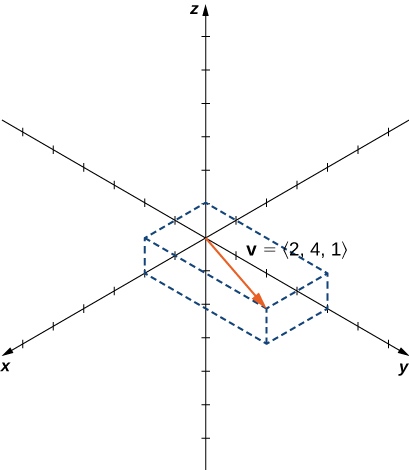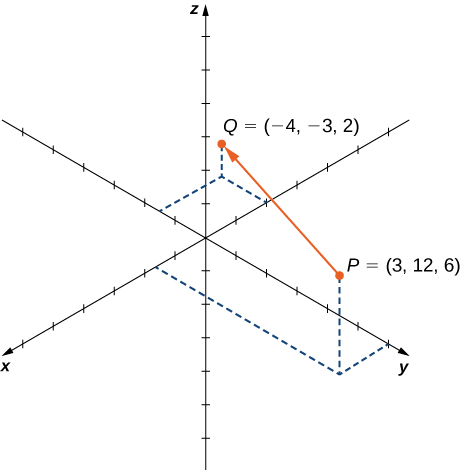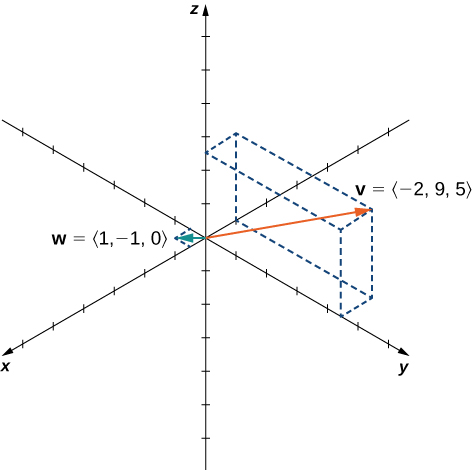# 2.2 Vectors in three dimensions  (Page 5/14)

 Page 5 / 14

Three-dimensional vectors can also be represented in component form. The notation $\text{v}=⟨x,y,z⟩$ is a natural extension of the two-dimensional case, representing a vector with the initial point at the origin, $\left(0,0,0\right),$ and terminal point $\left(x,y,z\right).$ The zero vector is $0=⟨0,0,0⟩.$ So, for example, the three dimensional vector $\text{v}=⟨2,4,1⟩$ is represented by a directed line segment from point $\left(0,0,0\right)$ to point $\left(2,4,1\right)$ ( [link] ).Vector v = ⟨ 2 , 4 , 1 ⟩ is represented by a directed line segment from point ( 0 , 0 , 0 ) to point ( 2 , 4 , 1 ) .

Vector addition and scalar multiplication are defined analogously to the two-dimensional case. If $\text{v}=⟨{x}_{1},{y}_{1},{z}_{1}⟩$ and $\text{w}=⟨{x}_{2},{y}_{2},{z}_{2}⟩$ are vectors, and $k$ is a scalar, then

$\text{v}+\text{w}=⟨{x}_{1}+{x}_{2},{y}_{1}+{y}_{2},{z}_{1}+{z}_{2}⟩\phantom{\rule{0.2em}{0ex}}\text{and}\phantom{\rule{0.2em}{0ex}}k\text{v}=⟨k{x}_{1},k{y}_{1},k{z}_{1}⟩.$

If $k=-1,$ then $k\text{v}=\left(-1\right)\text{v}$ is written as $\text{−}\text{v},$ and vector subtraction is defined by $\text{v}-w=v+\left(\text{−}\text{w}\right)=v+\left(-1\right)\text{w}.$

The standard unit vectors extend easily into three dimensions as well— $\text{i}=⟨1,0,0⟩,$ $\text{j}=⟨0,1,0⟩,$ and $\text{k}=⟨0,0,1⟩$ —and we use them in the same way we used the standard unit vectors in two dimensions. Thus, we can represent a vector in ${ℝ}^{3}$ in the following ways:

$\text{v}=⟨x,y,z⟩=x\text{i}+y\text{j}+z\text{k}.$

## Vector representations

Let $\stackrel{\to }{PQ}$ be the vector with initial point $P=\left(3,12,6\right)$ and terminal point $Q=\left(-4,-3,2\right)$ as shown in [link] . Express $\stackrel{\to }{PQ}$ in both component form and using standard unit vectors.The vector with initial point P = ( 3 , 12 , 6 ) and terminal point Q = ( −4 , −3 , 2 ) .

In component form,

$\begin{array}{cc}\hfill \stackrel{\to }{PQ}& =⟨{x}_{2}-{x}_{1},{y}_{2}-{y}_{1},{z}_{2}-{z}_{1}⟩\hfill \\ & =⟨-4-3,-3-12,2-6⟩=⟨-7,-15,-4⟩.\hfill \end{array}$

In standard unit form,

$\stackrel{\to }{PQ}=-7\text{i}-15\text{j}-4\text{k}.$

Let $S=\left(3,8,2\right)$ and $T=\left(2,-1,3\right).$ Express $\stackrel{\to }{ST}$ in component form and in standard unit form.

$\stackrel{\to }{ST}=⟨-1,-9,1⟩=\text{−}\text{i}-9\text{j}+\text{k}$

As described earlier, vectors in three dimensions behave in the same way as vectors in a plane. The geometric interpretation of vector addition, for example, is the same in both two- and three-dimensional space ( [link] ).

We have already seen how some of the algebraic properties of vectors, such as vector addition and scalar multiplication, can be extended to three dimensions. Other properties can be extended in similar fashion. They are summarized here for our reference.

## Rule: properties of vectors in space

Let $\text{v}=⟨{x}_{1},{y}_{1},{z}_{1}⟩$ and $\text{w}=⟨{x}_{2},{y}_{2},{z}_{2}⟩$ be vectors, and let $k$ be a scalar.

Scalar multiplication: $k\text{v}=⟨k{x}_{1},k{y}_{1},k{z}_{1}⟩$

Vector addition: $\text{v}+\text{w}=⟨{x}_{1},{y}_{1},{z}_{1}⟩+⟨{x}_{2},{y}_{2},{z}_{2}⟩=⟨{x}_{1}+{x}_{2},{y}_{1}+{y}_{2},{z}_{1}+{z}_{2}⟩$

Vector subtraction: $\text{v}-\text{w}=⟨{x}_{1},{y}_{1},{z}_{1}⟩-⟨{x}_{2},{y}_{2},{z}_{2}⟩=⟨{x}_{1}-{x}_{2},{y}_{1}-{y}_{2},{z}_{1}-{z}_{2}⟩$

Vector magnitude: $‖\text{v}‖=\sqrt{{x}_{1}{}^{2}+{y}_{1}{}^{2}+{z}_{1}{}^{2}}$

Unit vector in the direction of v: $\frac{1}{‖\text{v}‖}\text{v}=\frac{1}{‖\text{v}‖}⟨{x}_{1},{y}_{1},{z}_{1}⟩=⟨\frac{{x}_{1}}{‖\text{v}‖},\frac{{y}_{1}}{‖\text{v}‖},\frac{{z}_{1}}{‖\text{v}‖}⟩,$ if $\text{v}\ne 0$

We have seen that vector addition in two dimensions satisfies the commutative, associative, and additive inverse properties. These properties of vector operations are valid for three-dimensional vectors as well. Scalar multiplication of vectors satisfies the distributive property, and the zero vector acts as an additive identity. The proofs to verify these properties in three dimensions are straightforward extensions of the proofs in two dimensions.

## Vector operations in three dimensions

Let $\text{v}=⟨-2,9,5⟩$ and $\text{w}=⟨1,-1,0⟩$ ( [link] ). Find the following vectors.

1. $3\text{v}-2\text{w}$
2. $5‖\text{w}‖$
3. $‖5\text{w}‖$
4. A unit vector in the direction of $\text{v}$The vectors v = ⟨ −2 , 9 , 5 ⟩ and w = ⟨ 1 , −1 , 0 ⟩ .
1. First, use scalar multiplication of each vector, then subtract:
$\begin{array}{cc}\hfill 3\text{v}-2\text{w}& =3⟨-2,9,5⟩-2⟨1,-1,0⟩\hfill \\ & =⟨-6,27,15⟩-⟨2,-2,0⟩\hfill \\ & =⟨-6-2,27-\left(-2\right),15-0⟩\hfill \\ & =⟨-8,29,15⟩.\hfill \end{array}$
2. Write the equation for the magnitude of the vector, then use scalar multiplication:
$5‖\text{w}‖=5\sqrt{{1}^{2}+{\left(-1\right)}^{2}+{0}^{2}}=5\sqrt{2}.$
3. First, use scalar multiplication, then find the magnitude of the new vector. Note that the result is the same as for part b.:
$‖5\text{w}‖=‖⟨5,-5,0⟩‖=\sqrt{{5}^{2}+{\left(-5\right)}^{2}+{0}^{2}}=\sqrt{50}=5\sqrt{2}.$
4. Recall that to find a unit vector in two dimensions, we divide a vector by its magnitude. The procedure is the same in three dimensions:
$\begin{array}{cc}\hfill \frac{\text{v}}{‖\text{v}‖}& =\frac{1}{‖\text{v}‖}⟨-2,9,5⟩\hfill \\ & =\frac{1}{\sqrt{{\left(-2\right)}^{2}+{9}^{2}+{5}^{2}}}⟨-2,9,5⟩\hfill \\ & =\frac{1}{\sqrt{110}}⟨-2,9,5⟩\hfill \\ & =⟨\frac{-2}{\sqrt{110}},\frac{9}{\sqrt{110}},\frac{5}{\sqrt{110}}⟩.\hfill \end{array}$

how can chip be made from sand
are nano particles real
yeah
Joseph
Hello, if I study Physics teacher in bachelor, can I study Nanotechnology in master?
no can't
Lohitha
where we get a research paper on Nano chemistry....?
nanopartical of organic/inorganic / physical chemistry , pdf / thesis / review
Ali
what are the products of Nano chemistry?
There are lots of products of nano chemistry... Like nano coatings.....carbon fiber.. And lots of others..
learn
Even nanotechnology is pretty much all about chemistry... Its the chemistry on quantum or atomic level
learn
da
no nanotechnology is also a part of physics and maths it requires angle formulas and some pressure regarding concepts
Bhagvanji
hey
Giriraj
Preparation and Applications of Nanomaterial for Drug Delivery
revolt
da
Application of nanotechnology in medicine
has a lot of application modern world
Kamaluddeen
yes
narayan
what is variations in raman spectra for nanomaterials
ya I also want to know the raman spectra
Bhagvanji
I only see partial conversation and what's the question here!
what about nanotechnology for water purification
please someone correct me if I'm wrong but I think one can use nanoparticles, specially silver nanoparticles for water treatment.
Damian
yes that's correct
Professor
I think
Professor
Nasa has use it in the 60's, copper as water purification in the moon travel.
Alexandre
nanocopper obvius
Alexandre
what is the stm
is there industrial application of fullrenes. What is the method to prepare fullrene on large scale.?
Rafiq
industrial application...? mmm I think on the medical side as drug carrier, but you should go deeper on your research, I may be wrong
Damian
How we are making nano material?
what is a peer
What is meant by 'nano scale'?
What is STMs full form?
LITNING
scanning tunneling microscope
Sahil
how nano science is used for hydrophobicity
Santosh
Do u think that Graphene and Fullrene fiber can be used to make Air Plane body structure the lightest and strongest. Rafiq
Rafiq
what is differents between GO and RGO?
Mahi
what is simplest way to understand the applications of nano robots used to detect the cancer affected cell of human body.? How this robot is carried to required site of body cell.? what will be the carrier material and how can be detected that correct delivery of drug is done Rafiq
Rafiq
if virus is killing to make ARTIFICIAL DNA OF GRAPHENE FOR KILLED THE VIRUS .THIS IS OUR ASSUMPTION
Anam
analytical skills graphene is prepared to kill any type viruses .
Anam
Any one who tell me about Preparation and application of Nanomaterial for drug Delivery
Hafiz
what is Nano technology ?
write examples of Nano molecule?
Bob
The nanotechnology is as new science, to scale nanometric
brayan
nanotechnology is the study, desing, synthesis, manipulation and application of materials and functional systems through control of matter at nanoscale
Damian
how did you get the value of 2000N.What calculations are needed to arrive at it
Privacy Information Security Software Version 1.1a
Good
can you provide the details of the parametric equations for the lines that defince doubly-ruled surfeces (huperbolids of one sheet and hyperbolic paraboloid). Can you explain each of the variables in the equations?

#### Get Jobilize Job Search Mobile App in your pocket Now!By OpenStaxBy Jonathan LongBy Eric CrawfordBy Jonathan LongBy Brooke DelaneyBy Madison ChristianBy Savannah ParrishBy Janet ForresterBy OpenStaxBy OpenStax